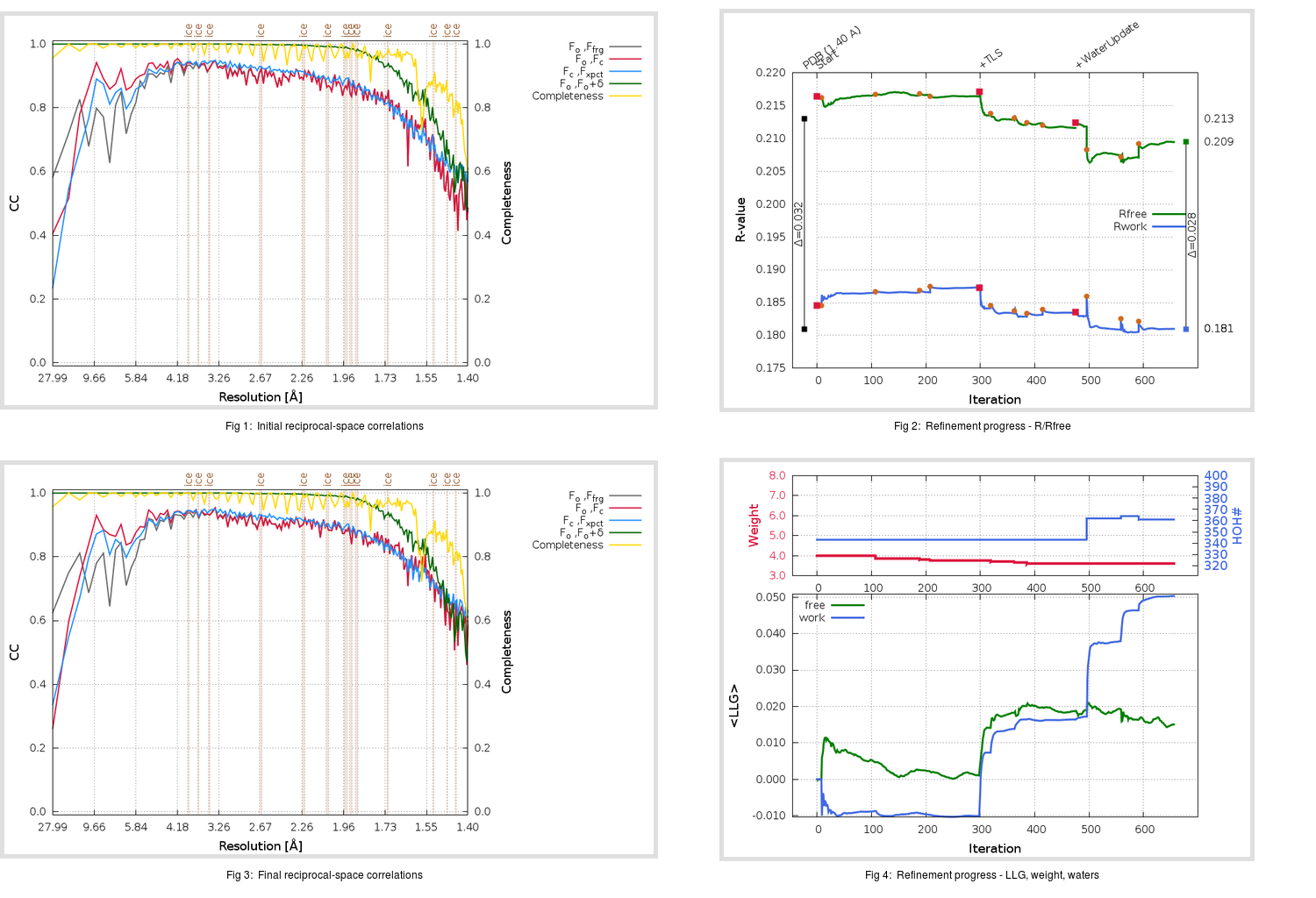Content:

## Deposited

` `
 Date deposited Date data collection Resolution R, Rfree 20200315 20200306 1.40 0.1800 0.2130

Molprobity (CCP4 7.0 version) summary:

```Ramachandran outliers =   0.33 %
favored =  98.01 %
Rotamer outliers      =   0.37 %
C-beta deviations     =     0
Clashscore            =   4.14
RMS(bonds)            =   0.0138
RMS(angles)           =   1.84
MolProbity score      =   1.20
Resolution            =   1.40
R-work                =   0.1800
R-free                =   0.2130
```

```Number of waters      =   343

<B> (all atoms) =   20.63 ( sd =    8.88 ) for       2788 non-hydrogen atoms
<B>   (protein) =   19.02 ( sd =    7.67 ) for       2416 non-hydrogen atoms
<B>     (water) =   31.53 ( sd =    9.27 ) for        343 non-hydrogen atoms
<B>    (others) =   24.60 ( sd =    9.04 ) for         29 non-hydrogen atoms

B min/max       (all non-hydrogen atoms) =    9.49 /   60.51
B min/max   (protein non-hydrogen atoms) =    9.49 /   60.51
B min/max     (water non-hydrogen atoms) =   11.84 /   57.81
B min/max     (other non-hydrogen atoms) =   18.66 /   35.61
```

## BUSTER (re-)refinement

` `

Molprobity (CCP4 7.0 version) summary:

```Ramachandran outliers =   0.33 %
favored =  98.68 %
Rotamer outliers      =   2.22 %
C-beta deviations     =     0
Clashscore            =   2.28
RMS(bonds)            =   0.0114
RMS(angles)           =   1.55
MolProbity score      =   1.27
Resolution            =   1.40
R-work                =   0.1810
R-free                =   0.2095
```

```Number of waters      =   361

<B> (all atoms) =   22.35 ( sd =    8.83 ) for       2806 non-hydrogen atoms
<B>   (protein) =   20.50 ( sd =    6.89 ) for       2416 non-hydrogen atoms
<B>     (water) =   34.27 ( sd =   10.68 ) for        361 non-hydrogen atoms
<B>    (others) =   25.87 ( sd =    9.22 ) for         29 non-hydrogen atoms

B min/max       (all non-hydrogen atoms) =    7.81 /  124.66
B min/max   (protein non-hydrogen atoms) =   10.48 /   57.38
B min/max     (water non-hydrogen atoms) =    7.81 /  124.66
B min/max     (other non-hydrogen atoms) =   20.33 /   34.63
```

Refinement progression:Results:

` `
 File Remark 5RFC_aB_refine.01_03_refine.pdb.gz exact refinement commands are in header 5RFC_aB_refine.01_03_refine.mtz.gz including original deposited data and several re-refinement map coefficients 5RFC_aB_refine.01_03_BUSTER_model.cif.gz including any non-standard compound restraints 5RFC_aB_refine.01_03_BUSTER_refln.cif.gz